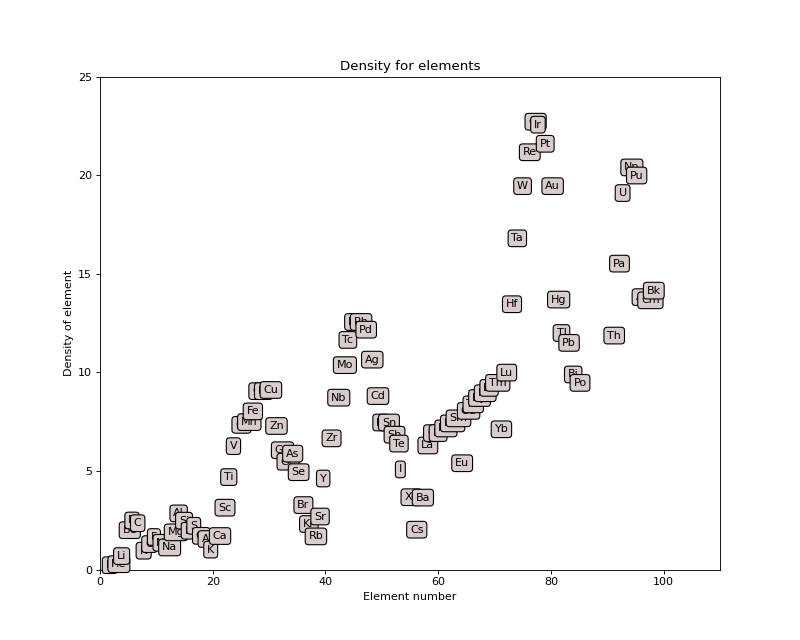$$\renewcommand\AA{\text{Å}}$$

# Density¶

## periodictable.density¶

• density, density_units (g·cm-3)
Densities for solids and liquids are given as specific gravities at 20° C unless other wise indicated by density_caveat. Densities for gaseous elements are given for the liquids at their boiling points. Missing data are represented by None.
• density_caveat
Comments on the density, if not taken in standard conditions.
• interatomic_distance, interatomic_distance_units (Å)
Interatomic distance estimated from element density.
• number_density, number_density_units (cm-3)
Number density estimated from mass and density.

Density for the isotope is computed assuming that the atomic spacing is the same as that for the element in the natural abundance.

>>> from periodictable import D, H
>>> print("H: %.4f, D: %.4f"%(H.density, D.density))
H: 0.0708, D: 0.1415
>>> print((D.density/H.density) / (D.mass/H.mass))
1.0


The following plot shows density for all elements:From the X-ray data book: http://xdb.lbl.gov/Section5/Sec_5-2.html

Data were taken mostly from . These values are reproduced in .

  Lide. D. R., Ed., CRC Handbook of Chemistry and Physics, 80th ed. (CRC Press, Boca Raton, Florida, 1999)
  The ILL Neutron Data Booklet, Second Edition.
periodictable.density.density(iso_el)

Element density for natural abundance. For isotopes, return the equivalent density assuming identical inter-atomic spacing as the naturally occuring material.

Parameters: iso_el : isotope or element Name of the element or isotope. density : float | g·cm-3
Reference:
ILL Neutron Data Booklet, original values from CRC Handbook of Chemistry and Physics, 80th ed. (1999).
periodictable.density.init(table, reload=False)
periodictable.density.interatomic_distance(element)

Estimated interatomic distance from atomic weight and density. The distance between isotopes is assumed to match that between atoms in the natural abundance.

Parameters: element : Element The element whose interatomic distance is to be calculated. distance : float | Å Estimated interatomic distance.

Interatomic distance is computed using:

$d = (m/(\rho_m N_A 10^{-24}))^{1/3}$

with units:

$(\rm (g\cdot mol^{-1}) / ( (g\cdot cm^{-3}) (atoms\cdot mol^{-1}) (10^{-8} cm\cdot \AA^{-1})^3))^{1/3} = \AA$
periodictable.density.number_density(element)

Estimate the number density from atomic weight and density. The density for isotopes is assumed to match that of between atoms in natural abundance.

Parameters: element : element Name of the element whose number density needs to be calculated. Nb : float | cm-3 Number density of a element.

Number density is computed using:

$d = N_A \frac{\rho}{m}$

with units:

$\rm (atoms\cdot mol^{-1}) (g\cdot cm^{-3}) / (g\cdot mol^{-1}) = atoms\cdot cm^{-3}$